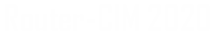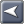﻿ Modals
Router-CIM Automation Suite

### Modals

Modals are defined as codes that only need to be output once to put a machine tool controller into a particular mode.  An example would be a typical cutting mode.  Once the controller is given a G01 code for cutting, it only needs X and Y codes for subsequent linear motions to be generated.  If the machine mode needs to be changed then another modal code is supplied.

Modals can also be referred to when addressing machine movements.  An example would be an X and Y motion.  Once an X or Y location is established by the controller, the X or Y value does not have to be addressed again until a change in the value occurs.  If the next motion of the machine has a different Y location and the same X location, only the Y location needs to be specified.

When a modal is referencing a machine mode, the modal is referred to as an ASCII modal because it is comparing letters.  When a modal is referencing a machine motion, the modal is referred to as a NUMERIC modal because it is comparing numbers.

A simple example of \$PP file entries would be:

G01;M#0=LINEAR

X#3.4;M#1=X

Y#3.4;M#1=Y

M#0 represents ASCII modals and M#1 represents NUMERIC modals.

A simple example of how these \$PP entries effect NC code is as follows:

 DXF file contains NC code produced Comments Text: "LINEAR" none G01 mode set Line: 0,0 to 1,1 N001G01X1.Y1. Output mode, X and Y motion Line: 1,1 to 2,1 N002X2. Output X motion ( Y the same)